# SJF Scheduling | SRTF | CPU Scheduling

## SJF Scheduling-

In SJF Scheduling,

• Out of all the available processes, CPU is assigned to the process having smallest burst time.
• In case of a tie, it is broken by FCFS Scheduling.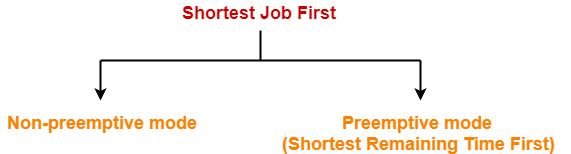• SJF Scheduling can be used in both preemptive and non-preemptive mode.
• Preemptive mode of Shortest Job First is called as Shortest Remaining Time First (SRTF).

• SRTF is optimal and guarantees the minimum average waiting time.
• It provides a standard for other algorithms since no other algorithm performs better than it.

• It can not be implemented practically since burst time of the processes can not be known in advance.
• It leads to starvation for processes with larger burst time.
• Priorities can not be set for the processes.
• Processes with larger burst time have poor response time.

## Problem-01:

Consider the set of 5 processes whose arrival time and burst time are given below-

 Process Id Arrival time Burst time P1 3 1 P2 1 4 P3 4 2 P4 0 6 P5 2 3

If the CPU scheduling policy is SJF non-preemptive, calculate the average waiting time and average turn around time.

## Solution-

### Gantt Chart-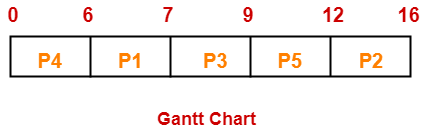Now, we know-

• Turn Around time = Exit time – Arrival time
• Waiting time = Turn Around time – Burst time

Also read- Various Times of Process

 Process Id Exit time Turn Around time Waiting time P1 7 7 – 3 = 4 4 – 1 = 3 P2 16 16 – 1 = 15 15 – 4 = 11 P3 9 9 – 4 = 5 5 – 2 = 3 P4 6 6 – 0 = 6 6 – 6 = 0 P5 12 12 – 2 = 10 10 – 3 = 7

Now,

• Average Turn Around time = (4 + 15 + 5 + 6 + 10) / 5 = 40 / 5 = 8 unit
• Average waiting time = (3 + 11 + 3 + 0 + 7) / 5 = 24 / 5 = 4.8 unit

## Problem-02:

Consider the set of 5 processes whose arrival time and burst time are given below-

 Process Id Arrival time Burst time P1 3 1 P2 1 4 P3 4 2 P4 0 6 P5 2 3

If the CPU scheduling policy is SJF preemptive, calculate the average waiting time and average turn around time.

## Solution-

### Gantt Chart-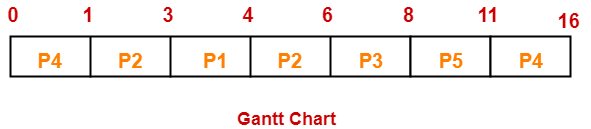Now, we know-

• Turn Around time = Exit time – Arrival time
• Waiting time = Turn Around time – Burst time

 Process Id Exit time Turn Around time Waiting time P1 4 4 – 3 = 1 1 – 1 = 0 P2 6 6 – 1 = 5 5 – 4 = 1 P3 8 8 – 4 = 4 4 – 2 = 2 P4 16 16 – 0 = 16 16 – 6 = 10 P5 11 11 – 2 = 9 9 – 3 = 6

Now,

• Average Turn Around time = (1 + 5 + 4 + 16 + 9) / 5 = 35 / 5 = 7 unit
• Average waiting time = (0 + 1 + 2 + 10 + 6) / 5 = 19 / 5 = 3.8 unit

## Problem-03:

Consider the set of 6 processes whose arrival time and burst time are given below-

 Process Id Arrival time Burst time P1 0 7 P2 1 5 P3 2 3 P4 3 1 P5 4 2 P6 5 1

If the CPU scheduling policy is shortest remaining time first, calculate the average waiting time and average turn around time.

## Solution-

### Gantt Chart-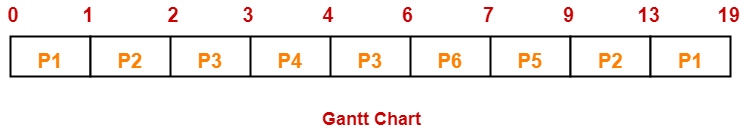Now, we know-

• Turn Around time = Exit time – Arrival time
• Waiting time = Turn Around time – Burst time

 Process Id Exit time Turn Around time Waiting time P1 19 19 – 0 = 19 19 – 7 = 12 P2 13 13 – 1 = 12 12 – 5 = 7 P3 6 6 – 2 = 4 4 – 3 = 1 P4 4 4 – 3 = 1 1 – 1 = 0 P5 9 9 – 4 = 5 5 – 2 = 3 P6 7 7 – 5 = 2 2 – 1 = 1

Now,

• Average Turn Around time = (19 + 12 + 4 + 1 + 5 + 2) / 6 = 43 / 6 = 7.17 unit
• Average waiting time = (12 + 7 + 1 + 0 + 3 + 1) / 6 = 24 / 6 = 4 unit

## Problem-04:

Consider the set of 3 processes whose arrival time and burst time are given below-

 Process Id Arrival time Burst time P1 0 9 P2 1 4 P3 2 9

If the CPU scheduling policy is SRTF, calculate the average waiting time and average turn around time.

## Solution-

### Gantt Chart-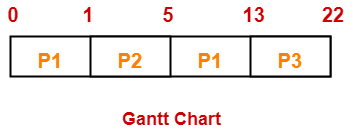Now, we know-

• Turn Around time = Exit time – Arrival time
• Waiting time = Turn Around time – Burst time

 Process Id Exit time Turn Around time Waiting time P1 13 13 – 0 = 13 13 – 9 = 4 P2 5 5 – 1 = 4 4 – 4 = 0 P3 22 22- 2 = 20 20 – 9 = 11

Now,

• Average Turn Around time = (13 + 4 + 20) / 3 = 37 / 3 = 12.33 unit
• Average waiting time = (4 + 0 + 11) / 3 = 15 / 3 = 5 unit

## Problem-05:

Consider the set of 4 processes whose arrival time and burst time are given below-

 Process Id Arrival time Burst time P1 0 20 P2 15 25 P3 30 10 P4 45 15

If the CPU scheduling policy is SRTF, calculate the waiting time of process P2.

## Solution-

### Gantt Chart-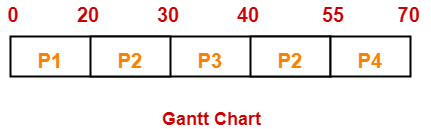Now, we know-

• Turn Around time = Exit time – Arrival time
• Waiting time = Turn Around time – Burst time

Thus,

• Turn Around Time of process P2 = 55 – 15 = 40 unit
• Waiting time of process P2 = 40 – 25 = 15 unit

## Implementation of Algorithm-

• Practically, the algorithm can not be implemented but theoretically it can be implemented.
• Among all the available processes, the process with smallest burst time has to be selected.
• Min heap is a suitable data structure where root element contains the process with least burst time.
• In min heap, each process will be added and deleted exactly once.
• Adding an element takes log(n) time and deleting an element takes log(n) time.
• Thus, for n processes, time complexity = n x 2log(n) = nlog(n)

To gain better understanding about SJF Scheduling,

Watch this Video Lecture

Next Article- Techniques to Predict Burst Time

Get more notes and other study material of Operating System.

Watch video lectures by visiting our YouTube channel LearnVidFun.

SummaryArticle Name
SJF Scheduling | SRTF | CPU Scheduling
Description
Shortest Job First or SJF Scheduling is a CPU Scheduling Algorithm that assigns CPU to the process with smallest burst time. Shortest Remaining Time First (SRTF) guarantees the minimal average waiting time and is optimal.
Author
Publisher Name
Gate Vidyalay
Publisher Logo